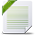# Decision Optimization

View Only

## How to use use the values of the decision variables indirectly to compute the value of the objective function using DOcplex.cp#### Philippe Laborie27 days ago• #### 1.  How to use use the values of the decision variables indirectly to compute the value of the objective function using DOcplex.cp

Posted 28 days ago

Hi,

I would appreciate your help regarding the following question. How can I use the values of the decision variables indirectly to compute the value of the objective function using DOcplex.cp. For clarity, I will explain the problem using the well-known house scheduling problem. Imagine that the cost of constructing the house depends on its duration. The longer the duration, the higher the cost. So, I would like to initiate the solution, put the values of the decision variables in a data frame, sum the column of the duration then calculate the cost (i.e. cost = 2*duration). The problem that the solver stops after getting the first feasible solution. I tried making a loop to overcome that problem as per the attached code, but it doesn't work.

``````import pandas
import time

from docplex.cp.model import CpoModel
from doopl.factory import *

# Create an OPL model from a .mod file
with create_opl_model(model="sched_sequence5.mod", data="sched_sequence5.dat") as opl:
# Generate the problem and solve it.
opl.run()
r = (opl.get_table('houses_initial'))

mdl = CpoModel(name='sched_sequence5')

mdl.import_model("sched_sequence5.cpo")

vars = mdl.get_all_variables()

Houses = []

for i in vars:
if "houses" in i.name:
Houses.append(i)

for i in vars:
if i.name == "cost":
cost_value = i

st = mdl.create_empty_solution()
st = mdl.start_search(SearchType='DepthFirst', Workers=1, TimeLimit=100)
final_solution = pandas.DataFrame(columns=['order', 'Start', 'End', 'Duration'])
duration = sum(r['duration'])
for msol in st:
for j in range(0, len(Houses)):
index2 = j
final_solution.loc[index2, 'order'] = j
final_solution.loc[index2, 'Start'] = msol.get_var_solution(Houses[j]).get_start()
final_solution.loc[index2, 'End'] = msol.get_var_solution(Houses[j]).get_end()
final_solution.loc[index2, 'Duration'] = msol.get_var_solution(Houses[j]).get_size()
duration = sum(final_solution['End'])
b = msol.print_solution()

``````

I am attaching the (.mod) and (.dat) files associated with that code. I would appreciate your inputs regarding this problem.

Mohamed

------------------------------
------------------------------

Attachment(s)sched_sequence5.dat   1 KB 1 versionsched_sequence5.mod   3 KB 1 version

• #### 2.  RE: How to use use the values of the decision variables indirectly to compute the value of the objective function using DOcplex.cp

Posted 27 days ago
Hi Mohamed,
What do you ant to do with the computed cost ? To reinfect it in the model so that the objective really is to minimize the cost that depends on the end times ?
Why don't you do it directly in the model ?
Is it because you cannot express the computation of the cost using an analytical expression in the model ? Then you should use blackbox expressions.

What you are doing with your current code is posting a new constraint "cost_value == 2*duration" at each iteration but this will be inconsistent in the model as you may post "cost_value == 100" in the first step and "cost_value == 80" in the next step.

Philippe

------------------------------
Philippe Laborie
------------------------------

• #### 3.  RE: How to use use the values of the decision variables indirectly to compute the value of the objective function using DOcplex.cp

Posted 26 days ago
Hi Philippe,

Thanks you for your kind reply. You are right. I cannot express the objective function analytically so, I am trying to build a kind of black-box function. That function takes the values from the decision variables then convert it into the objective function. I used the above example for simplicity but the actual problem I am working on is different.

I would like to ask if I can relate the durations of the decision intervals with the objective function as stated above?

Mohamed

------------------------------
------------------------------

• #### 4.  RE: How to use use the values of the decision variables indirectly to compute the value of the objective function using DOcplex.cp

Posted 26 days ago
Unfortunately, blackbox expressions are still not available in the Python API. You could do it in C++ of course.

In the absence of blackbox expression, the only thing you can do is a much weaker integration with the search if you can formulate in the model an approximation of the actual cost function, iterate on the improving solutions and evaluate the actual objective value on each solution and just keep the best one.

------------------------------
Philippe Laborie
------------------------------